메뉴 건너뛰기

### 5. Fibonacci Retracement

Fibonacci retracement?

The Fibonacci retracement was derived from a series of numbers discovered by the 13th century mathematician Leonardo Fibonacci. This mathematical relationship shows a sequence of numbers: 0%, 23.6%, 38.2%, 61.8%, 78.6%, 100%.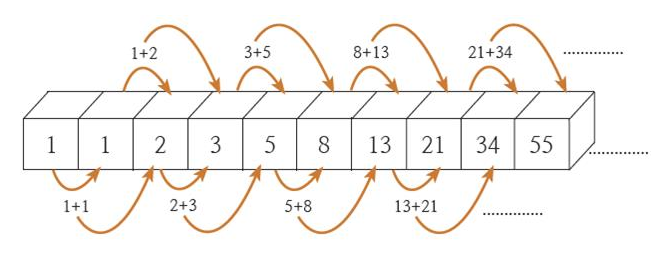What is an array?

An array is the sum of the leading and trailing numbers.

1,1,2,3,5,8,13,21,34,55,89,144 It goes like this.

So what about chart indicators? What is the Fibonacci retracement used as a concept?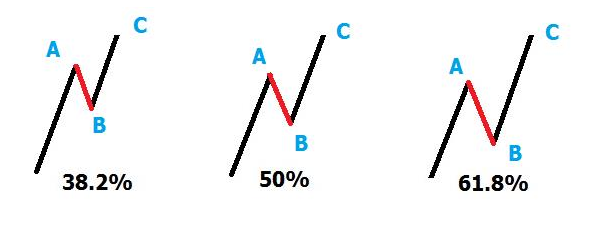Arrangement in Charts

Even on a rising chart, corrections always occur and take a break. Think of this as a retracement concept, and it is a term with a number by grafting the adjustment ratio to the Fibonacci ratio. 'Fibonacci retracement'

13/21,21/34,34/55 The leading number divided by the trailing number is 0.618.

21/55,34/89,55/144 The leading number divided by the trailing number is 0.382.

When expressed in %, the values ​​of 38.2% and 61.8% are obtained. The theory is that adding 50% to these two percentages will result in a retracement rate of 38.2%, 61,8%, and 50% when adjusted after an increase.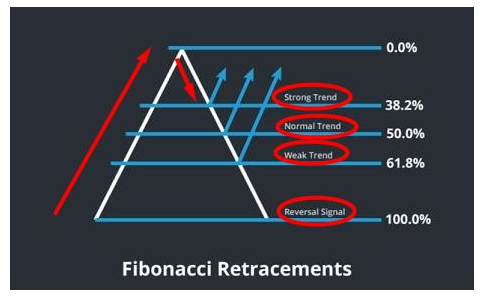This is used to predict the timing of a rebound after correction. If the retracement is short, the next rise is large, and if the retracement is large, the rise is generally low.

Fibonacci retracements are usually used in bull markets. I use the lows and highs in succession. Personally, I do not use the Fibonacci retracement as a lagging auxiliary indicator for trading. By using the Fibonacci retracement indicator, you can find a section with support and a section with resistance. In this way, you can set the timing of buying and selling.

The Fibonacci retracement indicator could be used for trading, but it is not necessarily the answer. If the Fibonacci retracement indicator could accurately predict it, all traders would have used it. Therefore, in order to know only what the Fibonacci retracement indicator is and use it for trading, it would be much more accurate to use other indicators together.

However, 0.5 and 0.618 are important values ​​when using the Fibonacci retracement. This is because this section (see image above) is most likely a strong support or resistance section. In other words, if you understand easily, it can be said that the points of 1/3 and 2/3 of the chart are 0.382 and 0.618 points.

Typically, these studies are used to predict levels of support and resistance through the relationship between Fibonacci numbers. The most used relationships are:

0.618 (61.8%): The Fibonacci number divided by the next number is about 0.618.

0.382 (38.2%): The Fibonacci number divided by the number occupying two postfixes is about 0.3820.

0.2360 (23.60%): The Fibonacci number divided by the number occupying three postfixes is approximately 0.2360.

0.764 (76.4%): The difference between 38.2% and 23.6% is obtained by adding 61.8%, i.e. 76.4% = 61.8% + (38.2% – 23.6%).

0.5 (50%): About half of the main flow, the average is 38.2% to 61.8%.

0.0 (0%): The start of the market movement.

1.9 (100%): End of market movement.

G
M
T

 언어를 감지갈리시아어구자라트어그루지야어그리스어네덜란드어네팔어노르웨이어덴마크어독일어라오어라트비아어라틴어러시아어루마니아어리투아니아어마라티어마오리어마케도니아어말레이어말라가시어말라얄람어몰타어몽골어몽어바스크어버마어베트남어벨로루시어벵골의보스니아어불가리아어세르비아어세부아노세소토어소말리아어순다어스와힐리어스웨덴어스페인어슬로바키아어슬로베니아어신할라어아랍어아르메니아어아이슬란드어아이티프랑스말아일랜드어아제르바이잔어아프리칸스어알바니아어에스토니아어에스페란토말영어요루바어우르두어우즈베크어우크라이나어웨일즈어이그보어이디시어이탈리아어인도네시아어일본어자바어줄루어중국어(간체)중국어(번체)체와어체코어카자흐어카탈로니아어칸나다어칸나다어크로아티아어타갈로그어타밀어타직어태국어터키어텔루구어펀자브어페르시아어포르투갈어폴란드어프랑스어핀란드어하우사어한국어헝가리어히브리어힌디어 갈리시아어구자라트어그루지야어그리스어네덜란드어네팔어노르웨이어덴마크어독일어라오어라트비아어라틴어러시아어루마니아어리투아니아어마라티어마오리어마케도니아어말레이어말라가시어말라얄람어몰타어몽골어몽어바스크어버마어베트남어벨로루시어벵골의보스니아어불가리아어세르비아어세부아노세소토어소말리아어순다어스와힐리어스웨덴어스페인어슬로바키아어슬로베니아어신할라어아랍어아르메니아어아이슬란드어아이티프랑스말아일랜드어아제르바이잔어아프리칸스어알바니아어에스토니아어에스페란토말영어요루바어우르두어우즈베크어우크라이나어웨일즈어이그보어이디시어이탈리아어인도네시아어일본어자바어줄루어중국어(간체)중국어(번체)체와어체코어카자흐어카탈로니아어칸나다어칸나다어크로아티아어타갈로그어타밀어타직어태국어터키어텔루구어펀자브어페르시아어포르투갈어폴란드어프랑스어핀란드어하우사어한국어헝가리어히브리어힌디어

음성 기능은 200자로 제한됨

 옵션 : 역사 : 피드백 : Donate 닫기
 2021년 해외코인거래소 순위 및 추천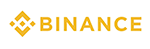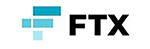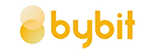거래량 11조(1위) 3.5조 9.5조 레버리지 최대100배 최대100배 최대125배 수수료 - 지정가 : 0.02%           - 시장가 : 0.04%(1위) - 지정가 : -0.025%                - 시장가 : 0.075% - 지정가 : -0.025%                  - 시장가 : 0.075% 거래방법 현물+선물+마진 선물+주식+FOREX 선물 회원가입 회원가입 회원가입 회원가입 할인코드 20% 할인 5% 할인 20% 할인